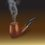# Dense subset of R

A set $$A$$ is said to be a dense subset of $$R$$ if $$\forall x,y\in R,x<y$$, $$\exists a\in A$$, such that $$x<a<y$$. My this note is to show that the set $$S=\left\{ m+n\sqrt { 2 } :m,n\in Z \right\}$$ is dense in $$R$$. For that we observe that $$S$$ satisfies the following properties:

i)Additivity: If $x,y\in S$, then $\left( x+y \right) \in S$.

ii)Homogeneity in $Z$: If $x\in S$ and $k\in Z$, then $kx\in S$

Now we observe that $\forall n\in Z$, $0\le \left( n\sqrt { 2 } -\left\lfloor n\sqrt { 2 } \right\rfloor \right) < 1$.

Also we note that $\forall m,n,m',n'\in Z$, $m+n\sqrt { 2 } =m'+n'\sqrt { 2 } \\ \Rightarrow m=m'\quad and\quad n=n'$

Now, let ${ s }_{ n }=\left( n\sqrt { 2 } -\left\lfloor n\sqrt { 2 } \right\rfloor \right) ,n\in Z$ Then $0\le { s }_{ n }< 1$ and ${ s }_{ n }\in S$. Also if $n\neq n'$, then ${ s }_{ n }\neq { s }_{ n' }$.Hence $\left\{ { s }_{ n }:n\in Z \right\}$ is an infinite subset of $S\cap [0,1)$.

Now we shall show that for any $\varepsilon >0$, $\exists s\in S$ such that $0. For that, given any $\varepsilon >0$, we partition $[0,1)$ into $k$ equal subintervals, each having size less than $\varepsilon$. Then, since $\left\{ { s }_{ n }:n\in Z \right\}$ is an infinite subset of $S\cap [0,1)$, so at least one subinterval must contain two distinct elements of $S\cap [0,1)$, say ${ s }_{ n },{ s }_{ n' }$. Without loss of generality, let ${ s }_{ n }<{ s }_{ n' }$. Then obviously $0<{ s }_{ n' }-{ s }_{ n }<\varepsilon$. Now, from the aforesaid properties i) and ii) of $S$, we have that $\left( { s }_{ n' }-{ s }_{ n } \right) =s\in S$ and hence $0. This shows that for any $\varepsilon >0$, $\exists s\in S$ such that $0.

Now let $\varepsilon >0$ be a real to be chosen such that $b-a>\varepsilon ,a,b\in R$ be given. Then it is trivial to show that $\exists {n}_{1}\in Z$ such that $a<{n}_{1}\varepsilon . Now we take $\varepsilon =\frac { b-a }{ 2 }$. Then $\exists s\in S$ such that $0. Hence, $\exists {n}_{2}\in Z$ such that $a<{n}_{2}s. Since by property ii), ${n}_{2}s\in S$,the property is proved.

There is another way to prove the above proposition. First prove that every proper subgroup of the additive group $R$ is either dense or cyclic in $R$. Then prove that the given subset $S$ of $R$ is a subgroup of $R$ that is not cyclic in $R$..hence it is dense in $R$.Note by Kuldeep Guha Mazumder
5 years, 7 months ago

This discussion board is a place to discuss our Daily Challenges and the math and science related to those challenges. Explanations are more than just a solution — they should explain the steps and thinking strategies that you used to obtain the solution. Comments should further the discussion of math and science.

When posting on Brilliant:

• Use the emojis to react to an explanation, whether you're congratulating a job well done , or just really confused .
• Ask specific questions about the challenge or the steps in somebody's explanation. Well-posed questions can add a lot to the discussion, but posting "I don't understand!" doesn't help anyone.
• Try to contribute something new to the discussion, whether it is an extension, generalization or other idea related to the challenge.

MarkdownAppears as
*italics* or _italics_ italics
**bold** or __bold__ bold
- bulleted- list
• bulleted
• list
1. numbered2. list
1. numbered
2. list
Note: you must add a full line of space before and after lists for them to show up correctly
paragraph 1paragraph 2

paragraph 1

paragraph 2

[example link](https://brilliant.org)example link
> This is a quote
This is a quote
    # I indented these lines
# 4 spaces, and now they show
# up as a code block.

print "hello world"
# I indented these lines
# 4 spaces, and now they show
# up as a code block.

print "hello world"
MathAppears as
Remember to wrap math in $$ ... $$ or $ ... $ to ensure proper formatting.
2 \times 3 $2 \times 3$
2^{34} $2^{34}$
a_{i-1} $a_{i-1}$
\frac{2}{3} $\frac{2}{3}$
\sqrt{2} $\sqrt{2}$
\sum_{i=1}^3 $\sum_{i=1}^3$
\sin \theta $\sin \theta$
\boxed{123} $\boxed{123}$

Sort by:

Your proof is very good in all respect. But I think there is some error at the very last paragraph. You have taken $\varepsilon = \frac {b-a} {2}$ so that $0 \lt \varepsilon \lt b - a.$ Then there exists $n_2 \in \Bbb Z$ such that $a \lt n_2 \varepsilon \lt b.$ Now since $(0, \varepsilon) \cap S \neq \varnothing$ there exists $s \in S$ such that $0 \lt s \lt \varepsilon.$ Now suppose $n_2 \geq 0$ then clearly $n_2 s \leq n_2 \varepsilon < b.$ How do I ascertain that $n_2 s \gt a\$? For instance we could take $a = \frac 1 3$ and $b = \frac 1 2.$ Then it is easy to check that $n_2 = 5.$ According to your claim we get $s \in S$ such that $0 \lt s \lt \frac {1} {12}.$ Then we have $n_2 s = 5 s \lt \frac {5} {12} \lt \frac {1} {2}.$ But how do I ascertain that $5 s \gt \frac 1 3.$ For that we need $s \gt \frac {1} {15}.$ How do you know that there is such an $s \in S$ with $\frac {1} {15} \lt s \lt \frac {1} {12}\$? Thus in all possible cases how do I ascertain that there exists such an $s \in S$ such that $n_2 s \gt a.$ Also if $n_2 \lt 0$ then multiplying $s$ by $n_2$ we have $n_2 s \gt n_2 \varepsilon.$ We have to deal with this fact too.

Hoping to get your response in this regard as soon as possible. Thanks in advance.

- 10 months ago